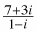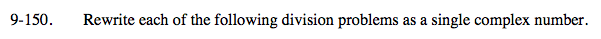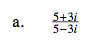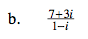Home > A2C > Chapter 9 > Lesson 9.3.2 > Problem9-150

9-150.
1. Rewrite each of the following division problems as a single complex number. Homework Help ✎

1.2.Multiply both the numerator and denominator by the denominator's conjugate.

$\frac{(5+3\textit{i})(5+3\textit{i})}{(5-3\textit{i})(5+3\textit{i})}$

$\frac{16+30\textit{i}}{34}$

$\frac{8}{17}+\frac{15}{17}i$See part (a).